## Curvilinear Coordinates

A general Metrichas a Line Element(1)

where Einstein Summation is being used. Curvilinear coordinates are defined as those with a diagonal Metric so that(2)

where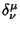is the Kronecker Delta. Curvilinear coordinates therefore have a simple Line Element(3)

which is just the Pythagorean Theorem, so the differential Vector is(4)

or(5)

where the Scale Factors are(6)

and(7)

Equation (5) may therefore be re-expressed as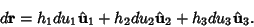(8)(9)

the Divergence is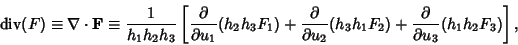(10)

and the Curl is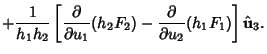(11)

Orthogonal curvilinear coordinates satisfy the additional constraint that(12)

Therefore, the Line Element is(13)

and the Volume Element is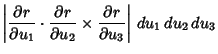(14)

where the latter is the Jacobian.

References

Arfken, G. Curvilinear Coordinates'' and Differential Vector Operators.'' §2.1 and 2.2 in Mathematical Methods for Physicists, 3rd ed. Orlando, FL: Academic Press, pp. 86-90 and 90-94, 1985.

Gradshteyn, I. S. and Ryzhik, I. M. Tables of Integrals, Series, and Products, 5th ed. San Diego, CA: Academic Press, pp. 1084-1088, 1980.

Morse, P. M. and Feshbach, H. Curvilinear Coordinates'' and Table of Properties of Curvilinear Coordinates.'' §1.3 in Methods of Theoretical Physics, Part I. New York: McGraw-Hill, pp. 21-31 and 115-117, 1953.